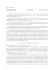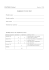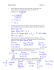## Transcription

```Outcome 2: Motion in Two Dimensions
Video notes:
•
•
•
•
Take handwritten or typed notes as you watch the video.
Notes should be kept in a bound notebook if handwriting or organized into a single document
(one per outcome) if typing.
Diligent effort into organization and neatness will benefit you. As the course goes by, you will
slowly be creating your own Physics 20 textbook. You can use these notes to review the material
while preparing for later assessments.
Completed videos notes for the outcome are required to earn a rewrite on the outcome test
Video note Checklist:
✔ Video Note
4.1
4.2
4.3
5.1
5.2
5.3
6.1
6.2
Complete before
Collinear and Perpendicular Vectors
Vector Components
Vectors at Angles
Horizontal Projectiles
Projectiles Launched at an Angle
Projectile Motion Examples
Relative Motion in 1D
Relative Motion in 2D
Concept Checks:
•
•
•
In order to be eligible for a rewrite for your outcome 1 test,
you must complete and self mark all concept checks for the outcome.
Complete each concept check without referring to your notes
or other resources. A calculator and a formula sheet are permitted.
Mark your concept check with a colored marking pen or pencil and write down corrections.
Concept Check Checklist
✔
Concept Check
✔
Completed Marked
Lesson 4
Lesson 5
Lesson 6
Practice Problems:
•
•
Practice Problems are not collected for marks. They are
intended to give you a chance to practice applying your understanding before small assessments
such as concept checks or larger assessments like the outcome tests.
While practice problems are optional, you will be more
successful if you have put significant effort into practicing your skills. Come by room 214 during
focus for assistance with practice problems.
Lesson 4 Practice Problems
Trig Review
1. Draw a right angle triangle and label all sides (a, b, c) and angles (A, B) (C = 90o). For angle
A write out the trigonometric functions: sine, cosine, and tangent. According to Pythagoras,
what is the length of the hypotenuse (side c)?
2. Find the number of degrees in each of the following angles:
(a) sin θ = 0.456
(27.1 o)
(b) sin θ = 0.748
(48.4 o)
(c) cos θ = 0.677
(47.4 o)
(d) cos θ = 0.224
(77.1 o)
(e) tan θ = 12.736
(85.5 o)
3. Find the values of the following:
a) sin 30, b) sin 45, c) sin 60, d) cos 30, e) cos 45, f) cos 60, g) tan 30, h) tan 45, i) tan 60
4. Given a right triangle with an hypotenuse length of 42 cm and θ=30 o. What are the lengths
of the opposite and adjacent sides? (21 cm, 36.4 cm)
5. For a particular right triangle θ=55o and the adjacent side with length 24 m, determine the
length of the hypotenuse. (41.8 m)
6. The opposite and adjacent sides of a right triangle are 12 cm and 16 cm respectively. What
is θ? What is the length of the hypotenuse? (36.9 o, 20 cm)
Video 4.1: Colinear and Perpendicular Vectors
For 1 to 3 do each of the following: (i) Draw a diagram (ii) Find distance (iii) Find displacement
1. An ant walks 20 cm [W] then 5 cm [N].
(25 cm, 20.6 cm @ 14 o N of W)
2. A mouse runs 30 m [W], 10 m [N], and then 20 m [W]. (60 m, 51 m @ 11 o N of W)
3. A hamster runs 60 cm [N], 30 cm [E], and then 90 cm [S].
of E)
(180 cm, 42.4 cm @ 45 o S
4. What is the displacement after a plane flies: 20 km [W], 50 km [S] and 60 km [E]?
(64.0 km @ 51.3 o S of E)
5. An object travels 858 m WEST then 297 m SOUTH. The time required for the journey was 13
minutes.
A. What was the total distance traveled? (1155 m)
B. What was the displacement of the object? (908 m @ 19 o S of W)
C. What was the average speed? (1.48 m/s)
6. An object travels 500 m south, 480 m east, 350 m north, 1650 m west, 390 m north, 590 m
east, and 190 m north. The entire trip required 3 hours and 21 minutes.
A. What was the resulting displacement of the object? (722 m @ 36 o N of W)
B. What was the average speed? (0.343 m/s)
7. A cyclist’s velocity is 10 m/s [245o]. Determine the x and y components of her velocity.
[vx = -4.2 m/s, vy = -9.1 m/s]
8. A hiker’s displacement is 15 km [40o E of N]. What is the north component of his
displacement? [11 km [N]]
9. Determine the distance travelled and the displacement for each of the following.
(a) Blading through Fish Creek Park in Calgary takes you 5.0 km [W], 3.0 km [N], 2.0 km
[E], and 1.5 km [S]. [distance = 11.5 km, displacement = 3.4 km [27o N of W]]
(b) A swimmer travels in a northerly direction across a 500-m-wide lake. Once across, the
swimmer then travels 150 m east. [distance = 650 m, displacement = 522 m [17o E of N]]
10.
Tourists on a jet ski move 1.20 km [55° N of E] and then 3.15 km [70° S of E].
Determine the jet ski’s displacement. [2.65 km [45o S of E]]
Video 4.2: Vector Components
1. Find the x and y components of the following displacements
a) 16.0 m [27o E of N]
Ans: [7.3 m east, 14.3 m north]
o
b) 10.0 m [33 S of E]
Ans:[8.39 m east, 5.44 m south]
o
c) 20.0 m [52.0 W of N]
Ans:[15.8 m west, 12.3 m north]
Video 4.3: Adding Vectors at Angles
Find the sum of the following sets of vectors (magnitude and direction).
1.
2.
3.
4.
5.
6.
7.
8.
0.389 m/s [38o N of E] and 0.934 m/s [10 o S of W]
4 N [east] and 8 N [north]
123.8 km/h [87 o N of W] and 67.89 km/h [22 o S of W]
5.6 m/s2 [Left] and 2.8 m/s2 [up]
36 cm [54 o S of E] and 24 cm [24 o S of W]
5.6 m [27 o N of E] and 2.3 m [E]
120 m/s [east] and 240 m/s [48 o S of W]
130 cm [46 o S of E] and 93 cm [2.0 o N of W]
9. Add the following displacement vectors
a) 3.0 m south and 4.0 m east [5.0 m 53o E of S]
b) 8.0 m west and 5.0 m north [9.4 m 32o N of W]
(0.618 m/s [7.2o N of W])
(9 N [63o N of E])
(120.4 km/h [55o N of W]])
(6.3 m/s2 [27o up from left])
(39 cm [89o S of W])
(7.7 m [19o N of E])
(183 m/s [77o S of W])
(90 cm [88o S of W])
Lesson 4 Concept Check
Add the following vectors. Draw a vector diagram.
1.2x1015 m [N] + 3.5x1016 m [S]
Draw the vector diagram to show the addition process and find the sum of the following vectors.
2.05 m [N] + 4 m [W]
Draw the vector triangle and find the x and y components for the following vector
1.67x1016 m/s [35o W of S]
A car travels 100.0 km [85o N of W] and then drives 87.0 km [13o E of N]. What is the car’s resulting
displacement? Show your work with diagrams and calculations.
80 m [65o E of N] + 67 m [45o N of E] + 10 m [W]
Lesson 5: Projectile Motion
Lesson 5 Practice Problems
5.1 Horizontal Projectiles
1. An object is thrown horizontally at a velocity of 10.0 m/s from the top of a 90.0 m building. Calculate
the distance from the base of the building that the object will hit the ground. [42.8 m]
2. An object is thrown horizontally at a velocity of 25.0 m/s from the top of a 1.50 x 102 m building.
Calculate the distance from the base of the building that the object will hit the ground. [1.38 x
102 m]
3. An object is thrown horizontally at a velocity of 20.0 m/s from the top of a cliff. If the object hit the
ground 48.0 m from the base of the cliff, how high is the cliff? [28.3 m]
4. An object is thrown horizontally from the top of a 85.0 m building. If the object hits the ground 67.8
m from the base of the building, what was the horizontal velocity of the object? [16.3 m/s]
5. An object is thrown horizontally at a velocity of 18.0 m/s from the top of a cliff. If the object hit the
ground 100.0 m from the base of the cliff, how high is the cliff. [151 m]
6. A person fires a bullet horizontally from a gun at the same instant that another bullet is dropped from
the same height as the rifle barrel. Discuss which bullet will hit the ground first.
7. A man is standing on the edge of the roof of a building that is 828.1 m high. If he throws an object
horizontally from the roof at 12 m/s, how far from the base of the building does the object land?
(1.6x102 m)
8. An airplane is flying level at 80.0 m above the ground with a speed of 350 km/h. The bombardier
wishes to drop food and medical supplies to hit a target on the ground. At what horizontal distance
from the target should the bombardier release the supplies? (383 m)
9. A car drives straight off the edge of a cliff that is 54 m high. The police at the scene of the accident
note that the point of impact is 130 m from the base of the cliff. How, fast was the car traveling when it
went over the cliff? (39 m/s)
5.2 Projectiles Launched at an Angle
1. Determine the height reached by a baseball if it is released with a velocity of 17.0 m/s [20o]. [1.72
m]
2. An object is thrown into the air with a velocity of 25.0 m/s at an angle of 32.0o to the horizontal.
How far will this object travel horizontally? [57.3 m]
3. An object is thrown from the ground into the air with a velocity of 20.0 m/s at an angle of 27.0o to
the horizontal. What is the maximum height reached by this object? [4.20 m]
4. An object is thrown from the ground into the air at an angle of 30.0o to the horizontal. If this object
reaches a maximum height of 5.75 m, at what velocity was it thrown? [21.2 m/s]
5. An object is thrown into the air with a velocity of 30.0 m/s at an angle of 35.0o to the horizontal.
How far will this object travel horizontally? [86.2 m]
6. An object is projected from the ground into the air at angle of 35.0o to the horizontal. If this object is
in the air for 9.26 s, at what velocity was it thrown? [79.2 m/s]
7. An object is thrown from the ground into the air at a velocity of 25.0 m/s at an unknown angle to the
horizontal. If this object travels 25.0 m horizontally in a time of 2.15 s, at what angle was this object
thrown? [62.3o]
8. An object is thrown into the air with a velocity of 30.0 m/s at an angle of 35.0o to the horizontal.
How far will this object travel horizontally? [86.2 m]
5.3 Extra Practice
1.
A cannon is fired at 30o above the horizontal with a velocity of 200 m/s from the edge of a 125 m
high cliff. Calculate where the cannonball lands on the level plain below. (3.7 x 103 m)
2.
A girl standing on the top of a roof throws a rock at 30 m/s at an angle of 30o below the horizontal.
If the roof is 50 m high, how far from the base of the building will the rock land? (52.3 m)
3.
A baseball is thrown at 30o above the horizontal with a speed of 49.0 m/s.
A.
B.
C.
D.
How long does it take for the ball to reach its maximum height? (2.50 s)
What is the maximum height? (30.6 m)
How long is the baseball in the air? (5.00 s)
What is the maximum range of the baseball? (212 m)
4.
An artillery gun is fired so that the shell has a vertical component of velocity of 210 m/s and a
horizontal component of 360 m/s. If the target is at the same level as the gun (a) how long will
the shell stay in the air and (b) how far down-range will the shell hit the target? (42.9 s, 15.4 km)
5.
If a stone is thrown at 60o above the horizontal with a speed of 196 m/s, what is the maximum
height and the range? (1469 m, 3391 m)
6.
From the top of a 10 m tower, a cannon launches a ball with an initial velocity of 45 m/s 10o above
the horizontal. How far does it travel in the horizontal direction?
7.
A ball is thrown with an initial velocity of 35 m/s 30o above the horizontal, off of a cliff. If it lands
230 m from the base of the cliff, how high is the cliff? (150 m)
8.
While crouching on the ground, you shoot an arrow at 27 m/s 10o above the horizontal. There is a
10 km/h wind traveling in the direction of the arrow’s horizontal motion. How high does the arrow
go? How far from you does it land? (1.12, 28 m)
9.
A girl standing on the top of a roof throws a rock at 30 m/s at an angle of 30o below the horizontal.
If the roof is 50 m high, how far from the base of the building will the rock land? (52 m)
10.
A quarterback throws a pass to a receiver, who catches it at the same height as the pass is thrown.
The initial velocity of the ball is 15.0 m/s, at an angle of 25.0o above the horizontal. How far away
Lesson 5 Concept Check
1. A projectile is launched off a 450 m cliff with an initial horizontal velocity of 75 m/s. What is the
speed of the projectile when it hits the ground?
2. A penny is kicked off the top of the Burj Khalifa building in Dubai with a horizontal velocity of 15
m/s. This is the tallest building in the world at 828 m. How far from the base of the building does
it land?
3. A soccer ball is kicked from the ground at 25 m/s [35o above the horizontal]. What is the
maximum height that the soccer ball reaches?
4. An object is launched off a 115 m cliff at a velocity of 65 m/s [35o
above the horizontal]. How far from the base of the cliff does it land?
5. A ball is launched at an angle off a 600 m high building. It lands 130 m from the base of the
building with a velocity of 700 m/s at 30o below the horizontal. What was the initial velocity of the
ball?
Lesson 6: Relative Motion
Lesson 6 Practice Problems
6.1 Relative Motion in 1D
1.
There are four animals in a race (the number in brackets indicates their top speeds); a
tortoise (0.2 m/s), a chicken (1.5 m/s), a rabbit (5.0 m/s) and a dog (7.0 m/s).
What is the velocity of:
A. The ground relative to the chicken? (1.5 m/s [left])
B. The rabbit relative to the dog?
(2.0 m/s
[left])
C. The tortoise relative to the chicken? (1.3 m/s
[left])
D. The ground relative to the rabbit? (5.0 m/s [left])
E. The dog relative to the ground? (7.0 m/s [left])
2.
F. The rabbit relative to the tortoise? (4.8 m/s [left])
G. The chicken relative to the tortoise? (1.3 m/s
[right])
H. The chicken relative to the dog? (5.5 m/s [left])
I. The dog relative to the dog? (0 m/s)
J. The tortoise relative to the dog? (6.8 m/s [left]
A train is going east with a speed of 30 m/s. Jim and Sam are passengers on the train and
they are moving away from each other. Jim is walking west with a speed of 1.5 m/s relative
to the train, while Sam is running east at 5.0 m/s relative to the train.
A. What is Jim's velocity relative to the ground? (28.5 m/s east)
B. What is Jim's velocity relative to Sam? (6.5 m/s west)
C. What is Sam's velocity relative to Jim? (6.5 m/s east)
D. What is Sam's velocity relative to the ground? (35.0 m/s east)
E. What is the train's velocity relative to Jim? (1.5 m/s east)
F. What is the train's velocity relative to Sam? (5.0 m/s west)
3.
A bus has a velocity of 25 m/s due south. A passenger walks to the back of the bus with a
speed of 1.0 m/s relative to the bus. What is the velocity (magnitude and direction) of the
passenger relative to a person standing on the ground? (24 m/s [S])
4.
Two cars, A and B, are traveling in the same direction, although car B is 186 m behind car A.
The speed of B is 24.4 m/s and the speed of A is 18.6 m/s. How much time does it take for B
to catch A? (32.1 s)
6.2 Relative Motion in 2D
1. A dog walks at 1.6 m/s on the deck of a boat that is traveling north at 7.6 m/s with respect to
the water.
(a) What is the velocity of the dog with respect to the water if it walks towards the bow?
(9.2 m/s [N])
(b) What is the velocity of the dog with respect to the water if it walks towards the stern?
(6.0 m/s [N])
(c) What is the velocity of the dog with respect to the water if it walks towards the east
rail, at right angles to the boat's keel? (7.8 m/s [78o N of E])
2.
An airplane with an airspeed of 400 km/h is flying south, but a west blowing wind of 86
km/h blows the plane off course. What was the actual velocity of the plane? (409 km/h [12o
W of S])
3.
Two people can paddle a canoe at 4.75 km/h in still water. A river has a 2.65 km/h current.
A. If the canoeists wish to paddle straight across the river, what angle should the keel of
the canoe make with the shoreline? (56o)
B.
If the river is 0.50 km wide, how much time is required to make the journey (in
minutes)? (7.6 min)
4.
The pilot of a ferry boat, with a cruising speed of 12 km/h, wishes to sail north from
Georgetown, Malaysia to Medan, Indonesia across the Strait of Malacca. If the strait has a
3.0 km/h current flowing west, what course should the pilot set to compensate for the
current? If the distance from Georgetown to Medan is 100 km, how long will the sailing
take? (14.5o E of N, 8.6 h)
5.
A pilot heads her plane with a velocity of 255 km/h north. If there is a strong 112 km/h
wind blowing east, what is the velocity of the plane in reference to the ground? (279 km/h
[23.7o E of N])
6.
A pilot wants to fly west. If the plane has an airspeed of 95 m/s and there is a 25 m/s wind
blowing north:
A. In what direction must she head the plane? (15.3o S of W)
B.
What will be her speed relative to the ground? (91.7 m/s)
C. How far will the plane go in 2.25 h? (743 km west)
7.
A 70 m wide river flows at 0.80 m/s. A girl swims across it at 1.4 m/s relative to the water.
(a) What is the least time she requires to cross the river? (50 s)
(b) How far downstream will she be when she lands on the opposite shore?
(40 m)
(c) At what angle to the shore would she have to aim, in order to arrive at a point directly
opposite the starting point? (55o)
(d) How long would the trip in part (c) take? (61 s)
8.
A yacht has a cruising speed of 45 km/h and it is headed east. If there is 20 km/h current
at 40o S of E, what is the actual velocity of the boat?
(61.7 km/h [12.0o S of E])
Lesson 6 Concept Check
1.
1.2 m/s [right]
1.5 m/s [left]
14 m/s [right]
vcat-rel-dog =
vtrex-rel-dog =
vdog-rel-dog =
vdog-rel-cat =
vtrex-rel-cat =
vcat-rel-cat =
vcat-rel-trex =
vdog-rel-trex =
vtrex-rel-trex =
2. A train is travelling at 15 m/s [west]. A t-rex runs along the roof of the train at 1.3 m/s [east] relative to
the train. What is the velocity of the t-rex relative to an observer on the ground?
3. a) A plane is traveling 150 m/s [S] when a strong wind sweeps moving at 125 m/s [E]. What is the
resulting velocity of the plane relative to the ground?
b) How far off course will the plane become in 2.3 h?
4. A t-rex can swim at 1.34 m/s in still water. He spots a tasty looking herbivore on
the north side of a river that is flowing at 0.75 m/s [W]. Being a very intelligent and
educated dinosaur, the t-rex pulls out a calculator and determines the exact angle at
which he should swim in order that he will arrive directly north at his meal. Calculate
the swimming angle of the t-rex.
```

### Vector Components Worksheet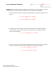### Throw a ball up and it will fall down 1) The force of gravity pulls the### Projectile Motion Worksheet (Case 1)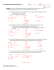### Solving Literal Equations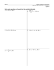### phys hw 9_23 - Science with Katie Dutton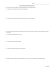### MAE 2600 (FS2014) Homework #18 Name: H18-1.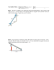### Speed, Velocity, and Acceleration Worksheet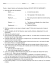### Homework II